##### Chemistry For Dummies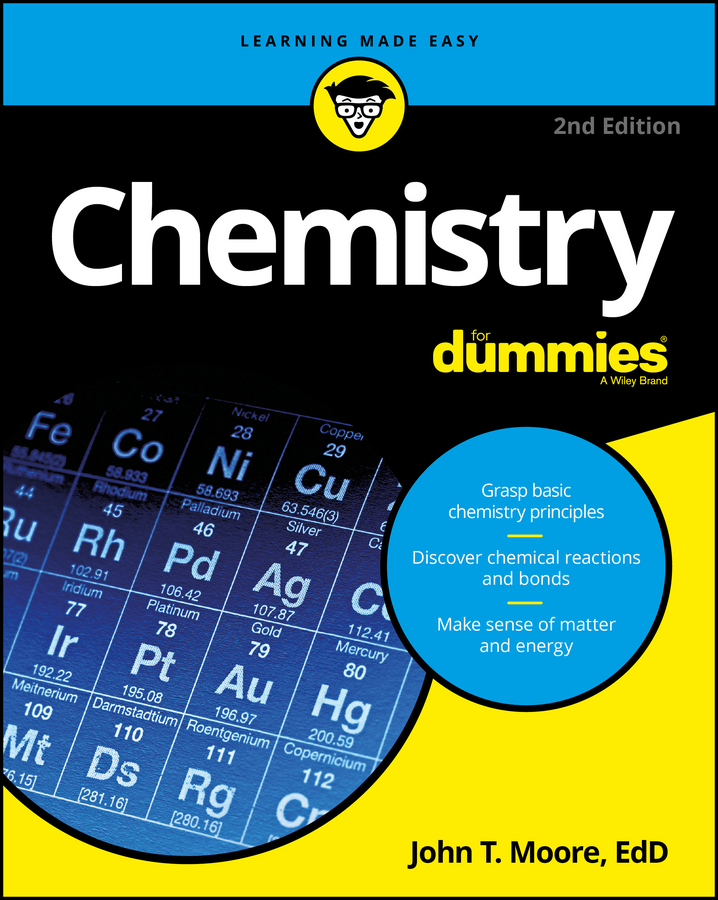There are several types of chemical formulas that you can use to represent chemical bonds. These include empirical formulas, molecular (or true) formulas, and structural formulas.

You can predict the formula of an ionic compound based on the loss and gain of electrons, to reach a noble gas configuration. However, you really can’t make that type of prediction with covalent compounds, because they can combine in many ways, and many different possible covalent compounds may result.

Most of the time, you have to know the formula of the molecule you’re studying. But you may have several different types of formulas, and each gives a slightly different amount of information.

## Empirical formula: Just the elements

The empirical formula indicates the different types of elements in a molecule and the lowest whole-number ratio of each kind of atom in the molecule. For example, suppose that you have a compound with the empirical formula: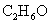Three different kinds of atoms are in the compound, C, H, and O, and they’re in the lowest whole-number ratio of 2 C to 6 H to 1 O. So the actual formula (called the molecular formula or true formula) may be any of the following, or another multiple of 2:6:1.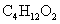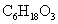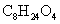## Molecular or true formula: Inside the numbers

The molecular formula, or true formula, tells you the kinds of atoms in the compound and the actual number of each atom.

You may determine, for example, that the following empirical formula is actually the molecular formula, too, meaning that there are actually two carbon atoms, six hydrogen atoms, and one oxygen atom in the compound:For ionic compounds, this formula is enough to fully identify the compound, but it’s not enough to identify covalent compounds. To write a formula that stands for the exact compound you have in mind, you often must write the structural formula instead of the molecular formula.

## Structural formula: Add the bonding pattern

The structural formula shows the elements in the compound, the exact number of each atom in the compound, and the bonding pattern for the compound. The electron-dot formula and Lewis formula are examples of structural formulas.

Look at the Lewis formulas presented in the following figure.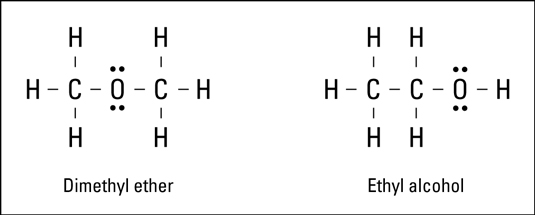Both compounds in the figure have two carbon atoms, six hydrogen atoms, and one oxygen atom. The difference is in the way the atoms are bonded, or what’s bonded to what. These are two entirely different compounds with two entirely different sets of properties:

• The formula on the left represents dimethyl ether. This compound is used in some refrigeration units and is highly flammable.

• The formula on the right represents ethyl alcohol, the drinking variety of alcohol.

Simply knowing the molecular formula isn’t enough to distinguish between the two compounds.

Compounds that have the same molecular formula but different structures are called isomers of each other. To identify the exact covalent compound, you need its structural formula.